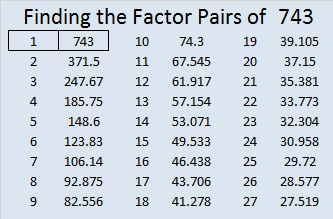# 743 and Level 5

• 743 is a prime number.
• Prime factorization: 743 is prime.
• The exponent of prime number 743 is 1. Adding 1 to that exponent we get (1 + 1) = 2. Therefore 743 has exactly 2 factors.
• Factors of 743: 1, 743
• Factor pairs: 743 = 1 x 743
• 743 has no square factors that allow its square root to be simplified. √743 ≈ 27.258026.How do we know that 743 is a prime number? If 743 were not a prime number, then it would be divisible by at least one prime number less than or equal to √743 ≈ 27.3. Since 743 cannot be divided evenly by 2, 3, 5, 7, 11, 13, 17, 19, or 23, we know that 743 is a prime number.

Here is today’s puzzle. It can be solved using logic as explained in the table at the end of the post.Print the puzzles or type the solution on this excel file: 12 Factors 2016-01-11

————————————-

Here is just a little more about the number 743:

743 is prime so it can be written as the sum of consecutive numbers only one way: 371 + 372 = 743.

743 is a palindrome in two bases:

• 616 BASE 11; note that 6(11²) + 1(11) + 6(1) = 743.
• 212 BASE 19; note that 2(19²) + 1(19) + 2(1) = 743.

————————————-